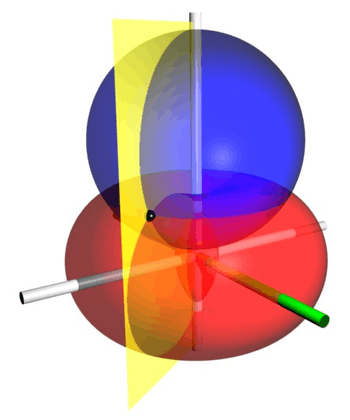# Bispherical coordinatesIllustration of bispherical coordinates, which are obtained by rotating a two-dimensional bipolar coordinate system about the axis joining its two foci. The foci are located at distance 1 from the vertical z-axis. The red self-intersecting torus is the σ=45° isosurface, the blue sphere is the τ=0.5 isosurface, and the yellow half-plane is the φ=60° isosurface. The green half-plane marks the x-z plane, from which φ is measured. The black point is located at the intersection of the red, blue and yellow isosurfaces, at Cartesian coordinates roughly (0.841, -1.456, 1.239).

Bispherical coordinates are a three-dimensional orthogonal coordinate system that results from rotating the two-dimensional bipolar coordinate system about the axis that connects the two foci. Thus, the two fociandin bipolar coordinates remain points (on the-axis, the axis of rotation) in the bispherical coordinate system.

## Definition

The most common definition of bispherical coordinatesiswhere thecoordinate of a pointequals the angleand thecoordinate equals the natural logarithm of the ratio of the distancesandto the foci### Coordinate surfaces

Surfaces of constantcorrespond to intersecting tori of different radiithat all pass through the foci but are not concentric. The surfaces of constantare non-intersecting spheres of different radiithat surround the foci. The centers of the constant-spheres lie along the-axis, whereas the constant-tori are centered in theplane.

### Inverse formulae

The formulae for the inverse transformation are:whereand### Scale factors

The scale factors for the bispherical coordinatesandare equalwhereas the azimuthal scale factor equalsThus, the infinitesimal volume element equalsand the Laplacian is given byOther differential operators such asandcan be expressed in the coordinatesby substituting the scale factors into the general formulae found in orthogonal coordinates.

## Applications

The classic applications of bispherical coordinates are in solving partial differential equations, e.g., Laplace's equation, for which bispherical coordinates allow a separation of variables. However, the Helmholtz equation is not separable in bispherical coordinates. A typical example would be the electric field surrounding two conducting spheres of different radii.

## Bibliography

• Morse PM, Feshbach H (1953). Methods of Theoretical Physics, Part I. New York: McGraw-Hill. pp. 665666.
• Korn GA, Korn TM (1961). Mathematical Handbook for Scientists and Engineers. New York: McGraw-Hill. p. 182. LCCN 59014456.
• Zwillinger D (1992). Handbook of Integration. Boston, MA: Jones and Bartlett. p. 113. ISBN 0-86720-293-9.
• Moon PH, Spencer DE (1988). "Bispherical Coordinates (η, θ, ψ)". Field Theory Handbook, Including Coordinate Systems, Differential Equations, and Their Solutions (corrected 2nd ed., 3rd print ed.). New York: Springer Verlag. pp. 110112 (Section IV, E4Rx). ISBN 0-387-02732-7.